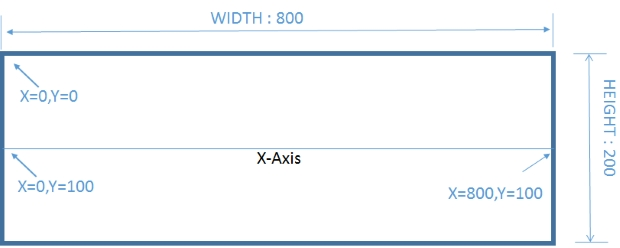# Drawing Sin & Cos Curve using PHP GD Support

X �Axis runs horizontally at the center for the image for which Y = 100.We will declare some variables.
``````\$width=800;  // Width of the Image Canvas
\$height=200; // Height of the canvas
\$steps=2;     // The Jump in value of X - Axis for each loop.
``````
Step: In the script the amount of movement of X axis in each loop. You can see we draw line between two coordinates and the value of 2nd coordinate is taken from the first.
``\$x2=\$x1+ \$steps``
Note that this value of the \$step determines the sharpness of the curves. Higher the value of step less will be the sharpness. Similarly with less number of steps more looping is required so sharpness of the curve increases.

## No of loops required.

To draw the curve we will use the loop and the loop will take one upper limit which is taken from this formula.
``for(\$i=1;\$i<=(\$width/\$steps);\$i++){``
As we start from left edge ( X=0 ) so number of steps required is total width divided by steps taken in each loop.

## How the curve is drawn

We used one for loop to draw lines between two coordinates. To draw this line we will use imageline function which takes two coordinates to draw line between them.

On next iteration of the loop, the 1st coordinate takes the values of 2nd coordinate of previous iteration of the loop.
For example in one loop the first coordinate is x1=20,y1=25 . Our 2nd Coordinate is x2=23,y2=27. IN the next loop we will have x1=23,y1=27, so the line will start from where we left at previous loop.
``\$x1=\$x2;``
We know that sin value varies between -1 and +1. SIN(90) = 1 and SIN(270)=-1.

So our curve will reach its peak at SIN(90) which should be within our top of the image. So we will use one multiplier to adjust the height of the curve.
``\$y1=(\$height/2)-number_format(sin(deg2rad(\$x1))*90,0);``
Our sin() function takes input in radian so we used deg2rad() function to convert degree value to radian. The math function number_format() is used to remove the decimal places. We have used 90 as multiplier factor here to scale the curve. If we use this value as 100 the top edge of the curve will match with top edge of the image. We kept it 90 to keep some gap. The complete code is here.
``````<?Php
\$width=800;  // Width of the Image Canvas
\$height=200; // Height of the canvas
\$steps=2;     // The Jump in value of X - Axis for each loop.

\$x1=0;

\$im = @ImageCreate (\$width, \$height)
or die ("Cannot Initialize new GD image stream");
\$background_color = ImageColorAllocate (\$im, 244, 244, 244); // Assign background color
\$text_color = ImageColorAllocate (\$im, 51, 51, 255); // text color is given
imageline ( \$im , 0 , \$height/2 , \$width , \$height/2 , 245 ); // X axis

for(\$i=1;\$i<=(\$width/\$steps);\$i++){

\$x2=\$x1+\$steps;

imageline ( \$im , \$x1 , \$y1 , \$x2 , \$y2 , \$text_color );

\$x1=\$x2;
}

ImagePng (\$im); // image displayed
?>``````

## Drawing COS curve

In your editor just search and replace sin with cos. Your cos curve is ready.
``````\$y1=(\$height/2)-number_format(cos(deg2rad(\$x1))*90,0);
\$x2=\$x1+\$steps;

``````
We can draw both curves SIN and COS in a single image. We have added thickness of the curve, text at X axis to the script. Here is the demo
``````<?Php
\$width=800;
\$height=200;
\$steps=2;     // The Jump in value of X - Axis for each loop.

\$x1=\$a1=0;

\$im = @ImageCreate (\$width, \$height)
or die ("Cannot Initialize new GD image stream");
\$background_color = ImageColorAllocate (\$im, 204, 204, 204); // Assign background color
\$text_color = ImageColorAllocate (\$im, 51, 51, 255); // text color is given
\$text_color1 = ImageColorAllocate (\$im, 0, 0, 0); // text color is given
imagesetthickness ( \$im , 2 );// manage thinkness of the curve.
imageline ( \$im , 0 , \$height/2 , \$width , \$height/2 , 245 ); // X axis

imagesetthickness ( \$im , 1 );// manage thinkness of the curve.

for(\$i=1;\$i<=(\$width/\$steps);\$i++){

\$x2=\$x1+\$steps;
\$a2=\$a1+\$steps;

//\$y2=30;

imageline ( \$im , \$x1 , \$y1 , \$x2 , \$y2 , \$text_color );
imageline ( \$im , \$a1 , \$b1 , \$a2 , \$b2 , \$text_color1 );
if(\$y2==100){ImageString(\$im,15,\$x2+5,\$y2,\$x2,\$text_color); } // Text on X -Axis

\$x1=\$x2;
\$a1=\$a2;
}

ImagePng (\$im); // image displayed
?>``````
Line Chart Different types of graphs by using Google Charts
Visitors Rating

GD functions in PHP for handling images & graphicsHow to check GD support in PHPHeader for an image in browserDrawing of graphic lines using GDPlotting line graph by drawing data from tableAdding vertical grid to line graphResizing and adding border to ImagesScript for Watermarking image on the fly using PHP GDScript to generate Captcha using GD with demoGetting Image coordinates ( vertical and horizontal ) of points inside a imagePage Hit counter script

Post your comments , suggestion , error , requirements etc here .